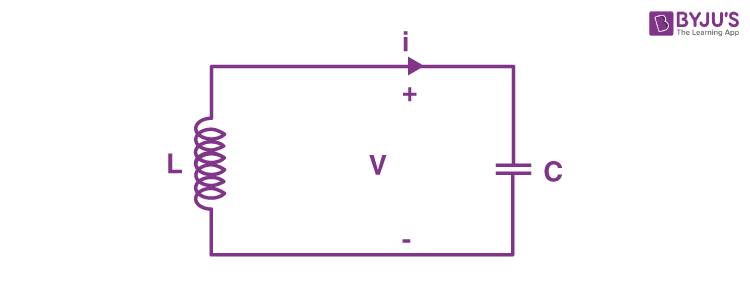# LC Circuit

An LC circuit is a type of an electric circuit that is made up of an inductor which is expressed by the letter L and a capacitor represented by the letter C. Here, both are connected in a single circuit. An LC circuit is also sometimes referred to as a tank circuit, resonant circuit, or tuned circuit. LC circuits act as major components in various electronic devices like radio equipment, in circuits like filters, oscillators, and tuners.LC circuit is a suitable model in many cases mainly because by using this type of circuit we can assume that there is no dissipation of energy even in if there is any resistance. However, if we were to be practical any implementation will involve loss because of the small electrical resistance in the connecting wires or components. This type of circuit is used because it can oscillate with minimum damping making the resistance as low as possible.  Nonetheless, most of the circuits work with some loss.

When an LC circuit oscillates at its natural resonant frequency, it can reserve electrical energy. The capacitor depending on the voltage it receives will store energy in the electric field (E) between its plates whereas an inductor depending on the current, it will accumulate energy in its magnetic field (B).

## Nomenclature

The di elemental LC circuit that we talked about in the above paragraphs is a basic example of an inductor-capacitor network. Moreover, it is also called a second-order LC circuit to differentiate it from highly complicated LC networks that have more capacitors and inductors. These LC networks that consist of more than two reactances can consist of several resonant frequencies.

A resonant frequency is defined as an undamped or natural frequency of a system. In the case of LC circuits, the resonant frequency is usually determined by the impedance L and capacitance C.

Meanwhile, the network order is an order of rational function which describes the network in complex frequency variables s. The order generally equals the number of L and C elements of the circuit and cannot exceed in any event.

## Applications or Uses of LC Circuit

The resonance effects of LC circuits have various applications which are important in communication systems and signal processing.

• LC circuits are utilized either to pick out or generate a signal at a certain frequency.
• Tuning radio transmitters and receivers are the most common application of tank circuits. For instance, when you tune a radio to some station, the LC circuits set a resonance for that carrier frequency.
• A parallel resonant circuit yields current magnification.
• A series resonant circuit yields voltage magnification.
• Both series and parallel resonant circuits are utilized in induction heating.
Test your knowledge on Lc Circuit